Updating search results...

# 8 Results

View
Selected filters:
• UT.MATH.III.A.REI.2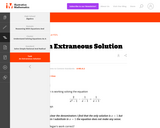Unrestricted Use
CC BY
Rating
0.0 stars

This is a task from the Illustrative Mathematics website that is one part of a complete illustration of the standard to which it is aligned. Each task has at least one solution and some commentary that addresses important aspects of the task and its potential use.

Subject:
Mathematics
Material Type:
Activity/Lab
Provider:
Illustrative Mathematics
Provider Set:
Illustrative Mathematics
Author:
Illustrative Mathematics
07/02/2018Unrestricted Use
CC BY
Rating
0.0 stars

This task provides a simple but interesting and realistic context in which students are led to set up a rational equation (and a rational inequality) in one variable, and then solve that equation/inequality for an unknown variable.

Subject:
Mathematics
Secondary Mathematics
Material Type:
Activity/Lab
Provider:
Illustrative Mathematics
Provider Set:
Illustrative Mathematics
Author:
Illustrative Mathematics
12/15/2012Unrestricted Use
CC BY
Rating
0.0 stars

This is a task from the Illustrative Mathematics website that is one part of a complete illustration of the standard to which it is aligned. Each task has at least one solution and some commentary that addresses important aspects of the task and its potential use.

Subject:
Mathematics
Material Type:
Activity/Lab
Provider:
Illustrative Mathematics
Provider Set:
Illustrative Mathematics
Author:
Illustrative Mathematics
07/02/2018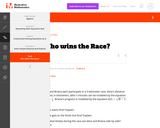Unrestricted Use
CC BY
Rating
0.0 stars

This is a task from the Illustrative Mathematics website that is one part of a complete illustration of the standard to which it is aligned. Each task has at least one solution and some commentary that addresses important aspects of the task and its potential use.

Subject:
Mathematics
Material Type:
Activity/Lab
Provider:
Illustrative Mathematics
Provider Set:
Illustrative Mathematics
Author:
Illustrative Mathematics
07/02/2018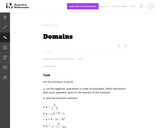Unrestricted Use
CC BY
Rating
0.0 stars

The purpose of this task to help students think about an expression for a function as built up out of simple operations on the variable, and understand the domain in terms of values for which each operation is invalid (e.g., dividing by zero or taking the square root of a negative number).

Subject:
Mathematics
Secondary Mathematics
Material Type:
Activity/Lab
Provider:
Illustrative Mathematics
Provider Set:
Illustrative Mathematics
Author:
Illustrative Mathematics
05/01/2012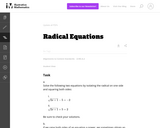Unrestricted Use
CC BY
Rating
0.0 stars

The purpose of the task is to show students a situation where squaring both sides of an equation can result in an equation with more solutions than the original one. The reason for this is that it is possible to have two unequal numbers whose squares are equal.

Subject:
Mathematics
Secondary Mathematics
Material Type:
Activity/Lab
Provider:
Illustrative Mathematics
Provider Set:
Illustrative Mathematics
Author:
Illustrative Mathematics
05/01/2012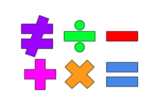Unrestricted Use
Public Domain
Rating
0.0 stars

This lesson is for a math classroom, but can be adapted to fit any grade, subject, or content.&nbsp;&nbsp;In this lesson, students will use an iPad and its features: Keynote, Pages, Garageband, Numbers, Presentation, and iMovie.&nbsp; Students will use 3 of those features/programs to create a video lesson consisting of several examples from a topic of their choice.&nbsp; This project is in place of a term final, so their chosen topic should be from their current term.Image citation: The image is one I created.&nbsp;&nbsp;

Subject:
Secondary Mathematics
Material Type:
Assessment
Author:
Tyrani Bevell
02/26/2022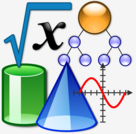Conditional Remix & Share Permitted
CC BY-NC
Rating
0.0 stars

Thumbnail photo:&nbsp;&nbsp;&quot;File:Nuvola Math and Inf.svg&quot;&nbsp;by&nbsp;Vojtěch Pikal&nbsp;is marked with&nbsp;CC0 1.0&nbsp;&nbsp;This is a lesson plan that can be used in any class and for any subject.&nbsp; The idea is to have the students review the content learned over the term, pick one of the concepts, and create a mini video lesson on the chosen concept.&nbsp; This is a project in place of a term final, but can be used at any time!I have tagged only a few math standards because this lesson applies to all content and all standards.

Subject:
Other
Material Type:
Lesson
Author:
Tyrani Bevell